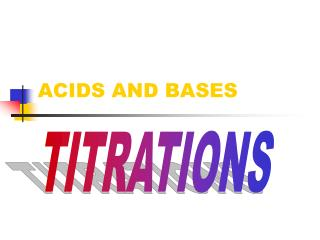DownloadDownload PresentationACIDS AND BASES

# ACIDS AND BASES

Download Presentation## ACIDS AND BASES

- - - - - - - - - - - - - - - - - - - - - - - - - - - E N D - - - - - - - - - - - - - - - - - - - - - - - - - - -
##### Presentation Transcript

1. ACIDS AND BASES TITRATIONS

2. Acid Base Titration A very accurate method to measure concentration. Acid + Base  Salt + Water H+ + OH- H2O Moles H+ = Moles OH-

3. Buret Solution with Indicator

4. Acid-Base Titrations • The amount of acid or base in a solution is determined by carrying out a neutralization reaction; an appropriate acid-base indicator (changes color in specific pH range) must be used to show when the neutralization is complete. • This process is called a TITRATION: the addition of a known amount of solution to determine the volume or concentration of another solution.

5. 3 steps… • Add a measured amount of an acid of unknown concentration to a flask. • Add an appropriate indicator to the flask (i.e. phenolphthalein) • Add measured amounts of a base of known concentration using a buret. Continue until the indicator shows that neutralization has occurred. This is called the end point of the titration.

6. Example: A 25-mL solution of H2SO4 is neutralized by 18 mL of 1.0 M NaOH using phenolphthalein as an indicator. What is the concentraion of the H2SO4 solution? • Equation: 2NaOH + H2SO4 2H2O + Na2SO4 • How many mol of NaOH are needed for neutralization?

7. Example: A 25-mL solution of H2SO4 is neutralized by 18 mL of 1.0 M NaOH using phenolphthalein as an indicator. What is the concentraion of the H2SO4 solution? • Equation: 2NaOH + H2SO4 2H2O + Na2SO4 • How many moles of H2SO4 were neutralized?

8. Example: A 25-mL solution of H2SO4 is neutralized by 18 mL of 1.0 M NaOH using phenolphthalein as an indicator. What is the concentraion of the H2SO4 solution? • Equation: 2NaOH + H2SO4 2H2O + Na2SO4 • Calculate the concentration of the acid:

9. Titration Curve: a graph showing how the pH changes as a function of the amount of added titrant in a titration. • Data for the graph is obtained by titrating a solution an measuring the pH after every drop of added titrant.

10. Titration Curve: a graph showing how the pH changes as a function of the amount of added titrant in a titration. • Equivalence point: the point on the curve where the moles of acid equal the moles of base; the midpoint of the steepest part of the curve (the most abrupt change in pH) is a good approximation of the equivalence point.

11. Titration Curve: a graph showing how the pH changes as a function of the amount of added titrant in a titration. • Knowledge of the equivalence point can then be used to choose a suitable indicator for a given titration; the indicator must change color at a pH that corresponds to the equivalence point.

12. Calculations of Titrations

13. 1) The Mole Method of Molarity: • Calculate the molarity of a sulfuric acid solution if 23.2 mL of it reacts with 0.212 g of Na2CO3. • H2SO4 + Na2CO3 Na2SO4 + CO2 + H2O

14. 2) Normality: • The normality (N) of a solution is defined as the (M) x (total positive oxidation)

15. HCl  H+ + Cl- H2SO4 2H+ + SO42- NaOH  Na+ + OH- Ba(OH)2 Ba2+ + 2OH- + ox = 1 + ox = 2 + ox = 1 + ox = 2 Examples:

16. Example: Calculate the molarity and normality of a solution that contains 34.2 g of Ba(OH)2 in 8.00 L of solution.

17. In titration problems, you can use this equation:

18. Example: 30.0 mL of 0.0750 N HNO3 required 22.5 mL of Ca(OH)2 for neutralization. Calculate the normality and molarity of the Ca(OH)2 solution.